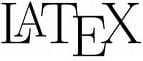0
Thanks

A few words of thanks would be greatly appreciated.

# LaTeX - Mathematical ExpressionsLaTeX possesses a highly developed mathematical mode that allows editing and display of mathematicals equations or formulas in a simple way.

There are two ways to access the math mode. The first way is to stay in the body text and enclose the mathematical formulas between the $character. When the$ character is doubled ($$) the formula will be detached from the body of the text. For example: "text  formul  text" gives the following result: text formula text "text$$ formula  text" gives the following result:
text
formula
text

Here is a list of useful commands especially designed for the mathematical mode:

Subscript and superscript

indices : $X_{indices}$
exponents : $X^{exponents}$

Note: if there is a symbol in superscript or subscript, then you can omit the braces.
• fractions: $\frac{numerator}{denominator}$
• root: $\sqrt{expression}$ for a square root, $\sqrt[n]{expression}$ for the nth root.

sum: $\displaystyle { \sum_{start condition}^{end condition}} expression of sum$
integrals: $\int_start^arrival f(x)dx$
limit: $\displaystyle {\lim_{variable \rightarrow (value being pointed to, by variable)}}f(x)$
To define matrices, we can either use the "array" environment or use the 'amsmath' package (you must then place the \usepackage{amsmath} command in the header of the document), using the following environments:
• matrix;
• pmatrix for a matrix enclosed in parentheses;
• bmatrix for a matrix surrounded by square brackets;
• vmatrix for a matrix enclosed by vertical lines;
• Vmatrix for a matrix enclosed by double vertical lines;

An example of a matrix:

$\begin{pmatrix} element1&element2 \\ element3&element4 \end{pmatrix}$


Here is a graphical result:Original document published on CommentcaMarche.net
0
Thanks

A few words of thanks would be greatly appreciated.